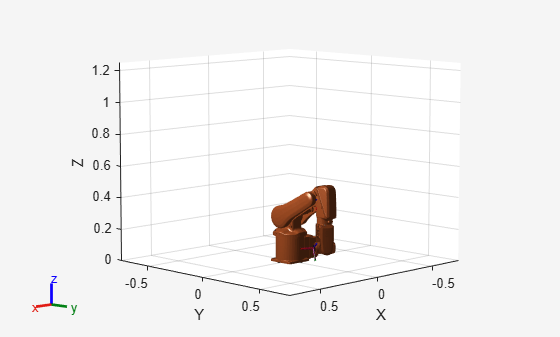# Simulate Joint-Space Trajectory Tracking in MATLAB

This example shows how to simulate the joint-space motion of a robotic manipulator under closed-loop control.

### Define Robot and Initial State

Load an ABB IRB-120T from the robot library using the `loadrobot` function.

```robot = loadrobot("abbIrb120T","DataFormat","column","Gravity",[0 0 -9.81]); numJoints = numel(homeConfiguration(robot));```

Define simulation parameters, including the time range over which the trajectory is simulated, the initial state as `[joint configuration; jointVelocity]`, and the joint-space set point.

```% Set up simulation parameters tSpan = 0:0.01:0.5; q0 = zeros(numJoints,1); q0(2) = pi/4; % Something off center qd0 = zeros(numJoints,1); initialState = [q0; qd0]; % Set up joint control targets targetJointPosition = [pi/2 pi/3 pi/6 2*pi/3 -pi/2 -pi/3]'; targetJointVelocity = zeros(numJoints,1); targetJointAcceleration = zeros(numJoints,1);```

Visualize the goal position.

`show(robot,targetJointPosition)````ans = Axes (Primary) with properties: XLim: [-1.5000 1.5000] YLim: [-1.5000 1.5000] XScale: 'linear' YScale: 'linear' GridLineStyle: '-' Position: [0.1300 0.1100 0.7750 0.8150] Units: 'normalized' Show all properties ```

### Model Behavior with Joint-Space Control

Using a `jointSpaceMotionModel` object, simulate the closed-loop motion of the model under a variety of controllers. This example compares a few of them. Each instance uses the `derivative` function to compute the state derivative. Here, the state is 2n-element vector `[joint configuration; joint velocity]`, where n is the number of joints in the associated `rigidBodyTree` object.

#### Computed-Torque Control

Computed-torque control uses an inverse-dynamics computation to compensate for the robot dynamics. The controller drives the closed-loop error dynamics of each joint based on a second-order response.

Create a `jointSpaceMotionModel` and specify the robot model. Set the `"MotionType"` to `"ComputedTorqueControl"`. Update the error dynamics using `updateErrorDynamicsFromStep` and specify the desired settling time and overshoot respectively. Alternatively, you can set the damping ratio and natural frequency directly in the object.

```computedTorqueMotion = jointSpaceMotionModel("RigidBodyTree",robot,"MotionType","ComputedTorqueControl"); updateErrorDynamicsFromStep(computedTorqueMotion,0.2,0.1);```

This motion model requires position, velocity, and acceleration to be provided.

`qDesComputedTorque = [targetJointPosition; targetJointVelocity; targetJointAcceleration];`

To view an example of this controller in practice in Simulink, see the Control LBR Manipulator Motion Through Joint Torque Commands example.

#### Independent Joint Control

With independent joint control, model each joint as a separate system that has a second-order tracking response. This type of model is an idealized behavior, and is best used when the response is slow, or when the dynamics will not have a significant impact on the resultant trajectory. In those cases, it will behave the same as computed-torque control, but with less computational overhead.

Create another `joinSpaceMotionModel` using the `"IndependentJointMotion"` motion type.

```IndepJointMotion = jointSpaceMotionModel("RigidBodyTree",robot,"MotionType","IndependentJointMotion"); updateErrorDynamicsFromStep(IndepJointMotion,0.2,0.1);```

This motion model requires position, velocity, and acceleration to be provided.

`qDesIndepJoint = [targetJointPosition; targetJointVelocity; targetJointAcceleration];`

#### Proportional-Derivative Control

Proportional-Derivative Control, or PD Control, combines gravity compensation with proportional and derivative gains. Despite the simpler nature relative to other closed-form models, the PD Controller can be stable for all positive gain values, which makes it a desirable option. Here, the PD Gains are set as n-by-n matrices, where n is the number of joints in the associated `rigidBodyTree` object. For this robot, n = 6. Additionally, PD Control does not require an acceleration profile, so its state vector is just a 2n-element vector of joint configurations and joint velocities.

```pdMotion = jointSpaceMotionModel("RigidBodyTree",robot,"MotionType","PDControl"); pdMotion.Kp = diag(300*ones(1,6)); pdMotion.Kd = diag(10*ones(1,6));```

This motion model requires position and velocity to be provided.

`qDesPD = [targetJointPosition; targetJointVelocity];`

### Simulate using an ODE Solver

The `derivative` function outputs the state derivative, which can be integrated using an ordinary differential equation (ODE) solver such as `ode45`. For each motion model, the ODE solver outputs a m-element column vector that covers `tspan` and a 2-by-m matrix of the 2n-element state vector at each instant in time.

Calculate the trajectory for each motion model, using the most appropriate ODE solver for each system.

```[tComputedTorque,yComputedTorque] = ode45(@(t,y)derivative(computedTorqueMotion,y,qDesComputedTorque),tSpan,initialState); [tIndepJoint,yIndepJoint] = ode45(@(t,y)derivative(IndepJointMotion,y,qDesIndepJoint),tSpan,initialState); [tPD,yPD] = ode15s(@(t,y)derivative(pdMotion,y,qDesPD),tSpan,initialState);```

### Plot Results

Once the simulation is complete, compare the results side-by-side. Each plot shows the joint position on the top, and velocity on the bottom. The dashed lines indicate the reference trajectories, while the solid lines display the simulated response.

```% Computed Torque Control figure subplot(2,1,1) plot(tComputedTorque,yComputedTorque(:,1:numJoints)) % Joint position hold all plot(tComputedTorque,targetJointPosition*ones(1,length(tComputedTorque)),'--') % Joint setpoint title('Computed Torque Motion: Joint Position') xlabel('Time (s)') ylabel('Position (rad)') subplot(2,1,2) plot(tComputedTorque,yComputedTorque(:,numJoints+1:end)) % Joint velocity title('Joint Velocity') xlabel('Time (s)') ylabel('Velocity (rad/s)')```In the following plot, use independent joint control to confirm that the computed torque motion behaves equivalently under some simplifying assumptions.

```% Independent Joint Motion figure subplot(2,1,1) plot(tIndepJoint,yIndepJoint(:,1:numJoints)) hold all plot(tIndepJoint,targetJointPosition*ones(1,length(tIndepJoint)),'--') title('Independent Joint Motion: Position') xlabel('Time (s)') ylabel('Position (rad)') subplot(2,1,2); plot(tIndepJoint,yIndepJoint(:,numJoints+1:end)) title('Joint Velocity') xlabel('Time (s)') ylabel('Velocity (rad/s)')```Finally, the PD Controller uses fairly aggressive gains to achieve similar rise times, but unlike the other approaches, the individual joints behave differently, since each joint and the associated bodies have slightly different dynamic properties that are not compensated by the controller.

```% PD with Gravity Compensation figure subplot(2,1,1) plot(tPD,yPD(:,1:numJoints)) hold all plot(tPD,targetJointPosition*ones(1,length(tPD)),'--') title('PD Controlled Joint Motion: Position') xlabel('Time (s)') ylabel('Position (rad)') subplot(2,1,2) plot(tPD,yPD(:,numJoints+1:end)) title('Joint Velocity') xlabel('Time (s)') ylabel('Velocity (rad/s)')```### Visualize the Trajectories as an Animation

To see what this behavior looks like in 3-D, the following example helper plots the robot motion in time. The third input is the number of frames between each sample.

`exampleHelperRigidBodyTreeAnimation(robot,yComputedTorque,1);``exampleHelperRigidBodyTreeAnimation(robot,yIndepJoint,1);``exampleHelperRigidBodyTreeAnimation(robot,yPD,1);`##### SupportGet trial now